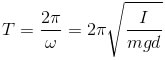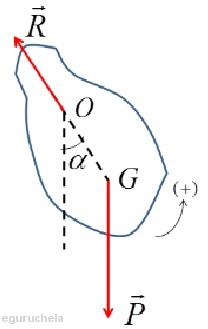﻿ All the formulas related to simple Harmonic Motion

# List of formulas related to simple Harmonic Motion

### Spring

K: spring constant (N/m), s: displacement (m), ω: angular velocity (rad/s), T: time period, m: mass of the object, A: amplitude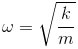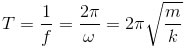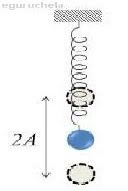### Springs in Series

k: spring constant of the system, T: time period of the system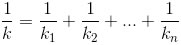### Springs in Parallel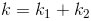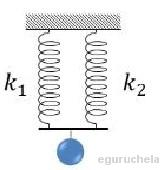### Simple Pendulum

ω: angular velocity (rad/s), T: time period , αo: angle at amplitude, A: amplitude, l: length of the pendulum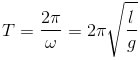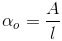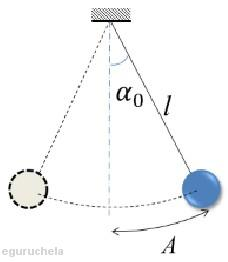### Motion Equations

S: displacement, α: angle, v: velocity, T: strength on the rod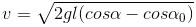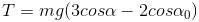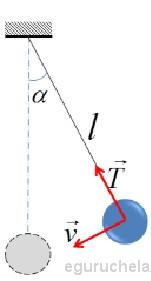### Energy

Ep: potential energy, Ek: kinetic energy, E: mechanical energy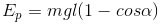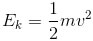### Change of Period Following The Change of The Temperature

Α: coefficient of linear expansion of the rod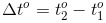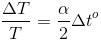### Change of Period Following The Change of The Height

h: height of the pendulum, R: earth’s radius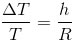### Pendulum

I: moment of inertia, ω: angular velocity, m: mass of bob, d: OG, T: time period